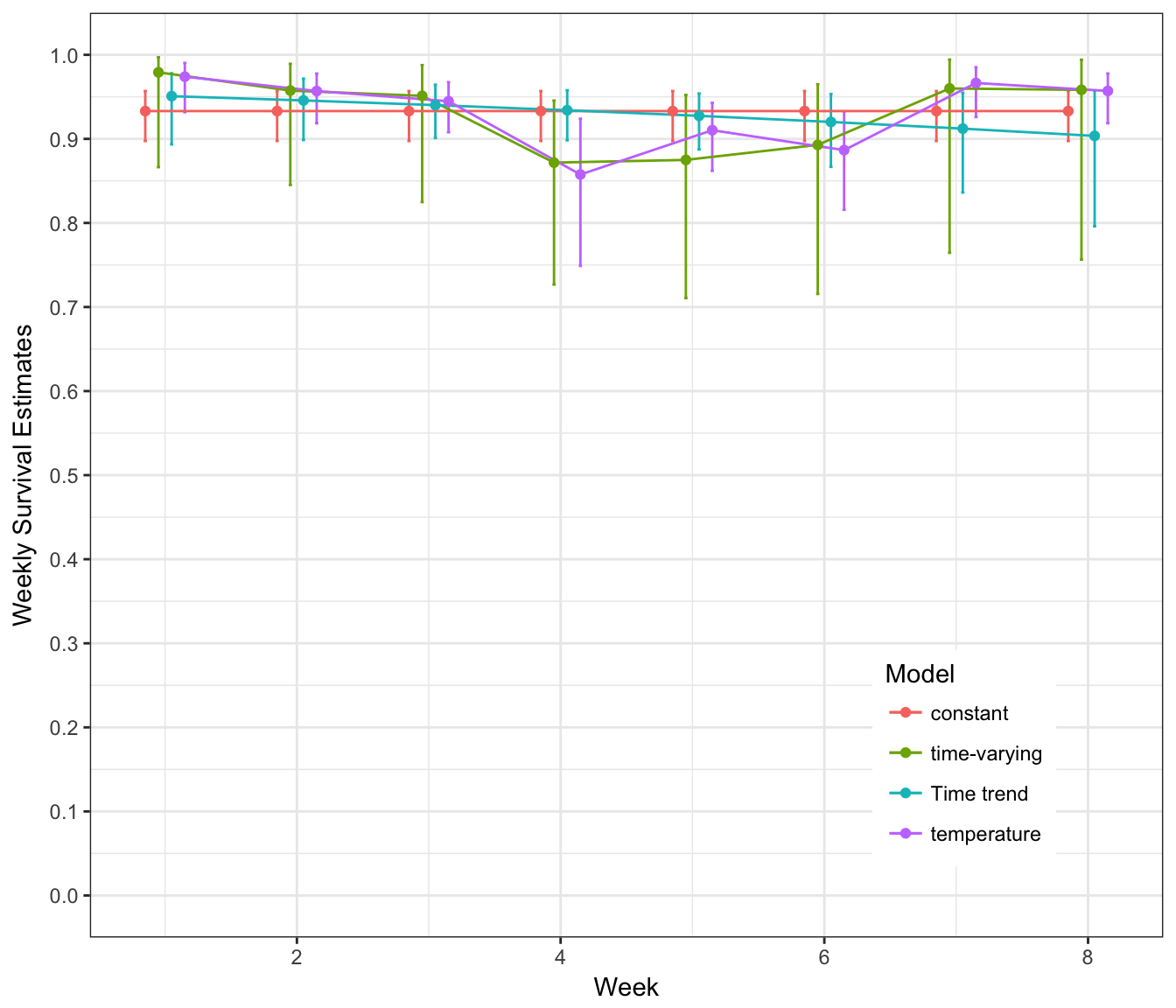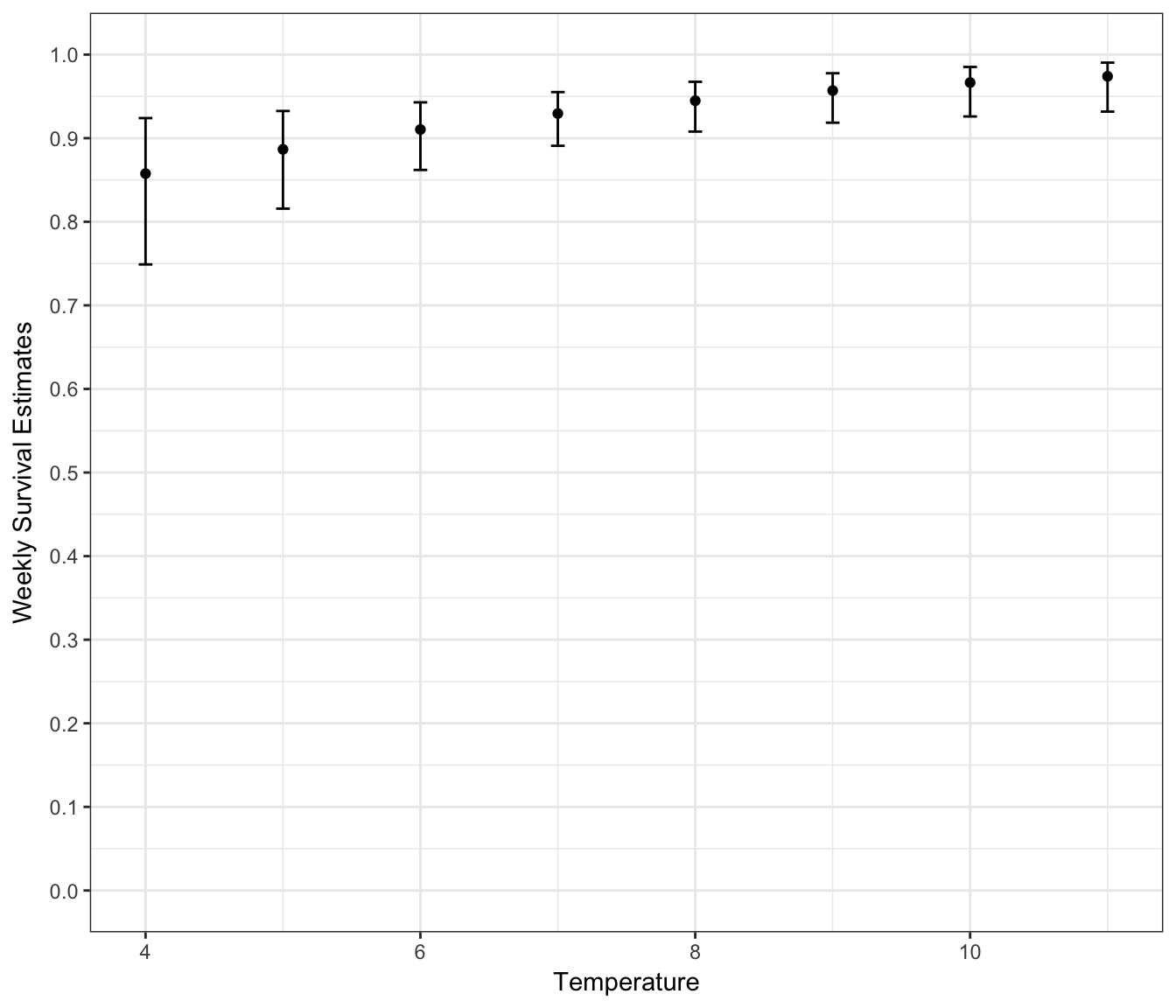In lab this week, we worked with data that were presented in a collapsed and very simple format. Here, we’ll work the data in a different format where the information is presented for 1 animal at a time. Here, we’ll work through the same models that you worked with directly in MARK. There are 2 things that are noteworthy about this exercise

• I made up the temperature data for the sake of the exercise
• In this data set, like the one that we used for the formal lab assignment, I killed 1 duck in the last week of the study, whereas in the original data set all ducks survived during the last week of the study, i.e., estimated survival rate was 1.0.

Here, the models are still run in Program MARK, but we will use the RMark interface to construct the models and view output. Once you learn MARK, doing analyses in RMark can save you some time and allow you to work with the estimates in some very useful ways. For example, you can use the many tools in R to graph the results, work with transformations of the estimates, and do a variety of other useful tasks. Working in RMark is not something I’ll require you to do for the course, but the material is here for you to explore.

### Bring in the data

library(RMark)
## This is RMark 2.2.4
##  Documentation available at http://www.phidot.org/software/mark/rmark/RMarkDocumentation.zip
library(ggplot2)

Blackduck = convert.inp("http://www.montana.edu/rotella/documents/502/ducknoco2.inp",
group.df = NULL, covariates = NULL)

head(Blackduck)
##                 ch freq
## 1 1100000000000000    1
## 2 1011000000000000    1
## 3 1011000000000000    1
## 4 1010000000000000    1
## 5 1010000000000000    1
## 6 1010110000000000    1

### Process the data & add temperature data

# Process the data for use in RMark
bduck.processed = process.data(Blackduck,
model = "Known",
begin.time = 0,
time.intervals = rep(1, 8))

# Create default design data
bduck.ddl = make.design.data(bduck.processed)

# Creat TIME variable that matches what we did in MARK using
#  values of 1, 2, ..., 8 rather that 0, 1, ..., 7, which
#  RMark uses by default
bduck.ddl$S$TIME = bduck.ddl$S$Time + 1

# Add occasion-specific data data for weekly temperature
bduck.ddl$S$temp = c(11, 9, 8, 4, 6, 5, 10, 9.01)

# examine a summary of the design data:
# note that 'time' is a factor & 'Time' is numeric
summary(bduck.ddl$S) ## par.index model.index group age time ## Min. :1.00 Min. :1.00 1:8 1 :1 1 :1 ## 1st Qu.:2.75 1st Qu.:2.75 2 :1 2 :1 ## Median :4.50 Median :4.50 3 :1 3 :1 ## Mean :4.50 Mean :4.50 4 :1 4 :1 ## 3rd Qu.:6.25 3rd Qu.:6.25 5 :1 5 :1 ## Max. :8.00 Max. :8.00 6 :1 6 :1 ## (Other):2 (Other):2 ## Age Time TIME temp ## Min. :1.00 Min. :0.00 Min. :1.00 Min. : 4.000 ## 1st Qu.:2.75 1st Qu.:1.75 1st Qu.:2.75 1st Qu.: 5.750 ## Median :4.50 Median :3.50 Median :4.50 Median : 8.500 ## Mean :4.50 Mean :3.50 Mean :4.50 Mean : 7.751 ## 3rd Qu.:6.25 3rd Qu.:5.25 3rd Qu.:6.25 3rd Qu.: 9.258 ## Max. :8.00 Max. :7.00 Max. :8.00 Max. :11.000 ##  ### Build function for creating models Here, we write a function that establishes which models are to be run in MARK. run.Blackduck = function() { # Define range of models for S S.dot = list(formula = ~ 1) # force use of identity matrix for time model by removing intercept S.time = list(formula = ~ -1 + time) S.TIME = list(formula = ~ TIME) S.temp = list(formula = ~ temp) # Create model list model.list = create.model.list("Known") # NOTE: to avoid having all the output for each model appear when you # call the function, add ', output=FALSE' after 'ddl=bduck.ddl' below. # Here, I don't do that so you can see the output for each model, # but this might not be desired if you have many models. bduck.results = mark.wrapper(model.list, data = bduck.processed, ddl = bduck.ddl) # Return model table and list of models return(bduck.results) } ### Run the models It’s worth noting that here, the output from each of the models appears in the console where it can be reviewed. One can also examine model-specific output in other ways as shown below. And, if you don’t want to see the output from every model appear in the output here, see the note above for the use of the “mark.wrapper” command where one has the option of suppressing the output. bduck.results = run.Blackduck() ## ## S.dot ## ## Output summary for Known model ## Name : S(~1) ## ## Npar : 1 ## -2lnL: 139.4719 ## AICc : 141.4861 ## ## Beta ## estimate se lcl ucl ## S:(Intercept) 2.635291 0.2374977 2.169795 3.100786 ## ## ## Real Parameter S ## 1 2 3 4 5 6 7 ## 0.9330986 0.9330986 0.9330986 0.9330986 0.9330986 0.9330986 0.9330986 ## 8 ## 0.9330986 ## ## S.temp ## ## Output summary for Known model ## Name : S(~temp) ## ## Npar : 2 ## -2lnL: 132.647 ## AICc : 136.6897 ## ## Beta ## estimate se lcl ucl ## S:(Intercept) 0.7512068 0.7191153 -0.6582593 2.1606729 ## S:temp 0.2611014 0.1029785 0.0592636 0.4629392 ## ## ## Real Parameter S ## 1 2 3 4 5 6 7 ## 0.9740002 0.9569389 0.9448004 0.857614 0.9103418 0.8866243 0.9665028 ## 8 ## 0.9570464 ## ## S.time ## ## Output summary for Known model ## Name : S(~-1 + time) ## ## Npar : 8 ## -2lnL: 132.0088 ## AICc : 148.5325 ## ## Beta ## estimate se lcl ucl ## S:time1 3.850148 1.0105825 1.8694061 5.830890 ## S:time2 3.113515 0.7226495 1.6971224 4.529908 ## S:time3 2.970414 0.7250110 1.5493927 4.391436 ## S:time4 1.916923 0.4789695 0.9781424 2.855703 ## S:time5 1.945910 0.5345225 0.8982460 2.993574 ## S:time6 2.120264 0.6110101 0.9226837 3.317843 ## S:time7 3.178054 1.0206208 1.1776370 5.178471 ## S:time8 3.135495 1.0215081 1.1333386 5.137650 ## ## ## Real Parameter S ## 1 2 3 4 5 6 7 8 ## 0.9791667 0.9574468 0.9512195 0.8717949 0.875 0.8928571 0.96 0.9583333 ## ## S.TIME ## ## Output summary for Known model ## Name : S(~TIME) ## ## Npar : 2 ## -2lnL: 138.5 ## AICc : 142.5427 ## ## Beta ## estimate se lcl ucl ## S:(Intercept) 3.0652343 0.5165318 2.052832 4.0776365 ## S:TIME -0.1034626 0.1045910 -0.308461 0.1015358 ## ## ## Real Parameter S ## 1 2 3 4 5 6 7 ## 0.9508169 0.9457466 0.9401865 0.9340962 0.9274337 0.9201553 0.9122159 ## 8 ## 0.9035698 ### Look at the model-selection table bduck.results ## model npar AICc DeltaAICc weight Deviance ## 2 S(~temp) 2 136.6897 0.00000 0.871727043 6.381189e-01 ## 1 S(~1) 1 141.4861 4.79639 0.079224173 7.463031e+00 ## 4 S(~TIME) 2 142.5427 5.85298 0.046711311 6.491102e+00 ## 3 S(~-1 + time) 8 148.5325 11.84281 0.002337473 -1.697087e-06 ### Working with output: Plotting It’s very common to want to plot results from various models of interest. Here, we put the estimates of “S” for each week from the ‘constant’,‘time’ ,‘Time-trend’, and ‘Temp’ models on a single plot. It’s pretty easy to do once you learn how to use R & RMark. The code below uses the ‘ggplot2’ package, which is well worth learning. # create data frames for estimates of real parameters from each model # with a row for each week's estimate and a covariate for week s.d = bduck.results$S.dot$results$real[, 1:4]
s.dot = cbind(s.d[rep(seq_len(nrow(s.d)), each = 8), ],
Week = 1:8,
Model = "constant")

s.t = cbind(bduck.results$S.time$results$real[, 1:4], Week = 1:8, Model = "time-varying") s.T = cbind(bduck.results$S.TIME$results$real[, 1:4],
Week = 1:8,
Model = "Time trend")

s.temp = cbind(bduck.results$S.temp$results$real[, 1:4], Week = 1:8, Model = "temperature") # store all the estimates in a data frame surv.ests = rbind(s.dot, s.t, s.T, s.temp) # Make plot # The errorbars overlapped, so use position_dodge to move them horizontally pd <- position_dodge(0.4) ggplot(surv.ests, aes(x = Week, y = estimate, color = Model)) + geom_errorbar(aes(ymin = lcl, ymax = ucl), width = 0.1, position = pd) + geom_line(position = pd) + geom_point(position = pd) + scale_y_continuous("Weekly Survival Estimates", limits = c(0, 1), breaks = seq(0, 1, by = 0.1)) + theme_bw() + # black and white theme theme(legend.justification = c(1, 0.1), legend.position = c(0.9, 0.1)) # Position legend in lower leftWe can plot the estimates of weekly survival for the temperature model against either week or temperature. Here, we first predict the survival rates for 8 different temperatures spaced across the range of values observed and then plot them. The way we do this below is to provide a design matrix with 8 rows and 2 columns as that’s what we used in building the model (check out the object ‘bduck.results$$S.temp$$design.matrix’ if you want to see it). Here, we present 8 nicely ordered temperature values (4, 5, …, 10, 11) so we can plot estimated survival against temperature. (If you wanted to use more than 8 values, you could create time-varying individual covariates that would be the same for all individuals for a given occasion but that would change across occasions. This is explained in detail by Jeff Laake in his RMark workshop notes. The idea is beyond where we are in the course, so we won’t go into that further now). # replace the original temp values with ordered range of 8 values # that cover the range of observed values S.temp.pred = compute.real(bduck.results$S.temp,
design = matrix(c(rep(1, 8), 4:11),
8, 2, byrow = F))
S.temp.pred$Temperature = 4:11 ggplot(S.temp.pred, aes(x = Temperature, y = estimate)) + geom_errorbar(aes(ymin = lcl, ymax = ucl), width = 0.1) + geom_point() + scale_y_continuous("Weekly Survival Estimates", limits = c(0, 1), breaks = seq(0, 1, by = 0.1)) + theme_bw()We can also use RMark’s model-averaging function to obtain estimates of weekly survival rates that are weighted averages of each model’s estimates where the weights are the AICc weights. For this study, consider what the estimates of weekly survival are for each model. Next, consider what the AICc weights are for each model. Finally, calculate a weighted average using that information. The model weights sum to 1.0, so the weighted average is simply the sum of each model’s estimate of a given week’s survival rate multiplied by the weight of support for that model, i.e., $$\hat{\bar{S_{i}}}=\sum_{i=1}^{n}\hat{S_{i}}*w_{i}$$. Here, the model-specific estimates of survival in week 1 were:(1) 0.933 for the S(.) model; (2) 0.979 for the S(time) model; (3) 0.951 for the S(Time-trend) model; and (4) 0.974 for the S(temp) model. The corresponding weights were 0.079, , , and 0.872. The weighted average takes into account (1) the model-selection uncertainty as well as (2) the model-specific uncertainty in the estimates. For this dataset and model set, estimates from the S(temp) model are heavily weighted, estimates from the S(time) model receive almost no weight, and estimates from the S(.) model and the S(Time-trend) model receive modest amounts of weight. S.weekly.averages=model.average(bduck.results,vcv=TRUE) s.mod.avg = S.weekly.averages$estimates[, 2:5]
s.mod.avg$Week = 1:8 s.mod.avg$Model = "Model-avg"

ggplot(s.mod.avg, aes(x = Week, y = estimate)) +
geom_errorbar(aes(ymin = lcl, ymax = ucl),
width = 0.1) +
geom_point() +
scale_y_continuous("Model-Averaged Weekly Survival Estimates",
limits = c(0, 1),
breaks = seq(0, 1, by = 0.1)) +
theme_bw()